## Determining the Eigenvectors of a Matrix

In order to determine the eigenvectors of a matrix, you must first determine the eigenvalues. Substitute one eigenvalue λ into the equation A x = λ x—or, equivalently, into ( A − λ I) x = 0—and solve for x; the resulting nonzero solutons form the set of eigenvectors of A corresponding to the selectd eigenvalue. This process is then repeated for each of the remaining eigenvalues.

Example 1: Determine the eigenvectors of the matrix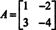In Example 1, the eigenvalues of this matrix were found to be λ = −1 and λ = −2. Therefore, there are nonzero vectors x such that A x = x (the eigenvectors corresponding to the eigenvalue λ = −1), and there are nonzero vectors x such that A x = −2 x (the eigenvectors corresponding to the eigenvalue λ = −2). The eigenvectors corresponding to the eigenvalue λ = −1 are the solutions of the equation A x = −x: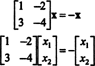This is equivalent to the pair of equationswhich simplifies to[Note that these equations are not independent. If they were independent, then only ( x 1, x 2) T = (0, 0) T would satisfy them; this would signal that an error was made in the determination of the eigenvalues. If the eigenvalues are calculated correctly, then there must be nonzero solutions to each system A x = λ x.] The equations above are satisfied by all vectors x = ( x 1, x 2) T such that x 2 = x 1. Any such vector has the form ( x 1, x 2) T. and is therefore a multiple of the vector (1, 1) T. Consequently, the eigenvectors of A corresponding to the eigenvalue λ = −1 are precisely the vectors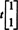where t is any nonzero scalar.

The eigenvectors corresponding to the eigenvalue λ = −2 are the solutions of the equation A x = −2 x:This is equivalent to the “pair” of equations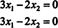Again, note that these equations are not independent. They are satisfied by any vector x = ( x 1, x 2) T that is a multiple of the vector (2, 3) T; that is, the eigenvectors of A corresponding to the eigenvalue λ = −2 are the vectors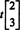where t is any nonzero scalar.

Example 2: Consider the general 2 x 2 matrix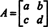Express the eigenvalues of A in terms of a, b, c, and d. What can you say about the eigenvalues if b = c (that is, if the matrix A is symmetric)?

Verify that the sum of the eigenvalues is equal to the sum of the diagonal entries in A.

Verify that the product of the eigenvalues is equal to the determinant of A.

What can you say about the matrix A if one of its eigenvalues is 0?

The solutions are as follows:

The eigenvalues of A are found by solving the characteristic equation, det ( A − λ I) = 0: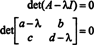The solutions of this equation—which are the eigenvalues of A—are found by using the quadratic formula: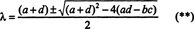The discriminant in (**) can be rewritten as follows:Therefore, if b = c, the discriminant becomes ( a − d) 2 + 4 b 2 = ( a − d) 2 + (2 b) 2. Being the sum of two squares, this expression is nonnegative, so (**) implies that the eigenvalues are real. In fact, it can be shown that the eigenvalues of any real, symmetric matrix are real.

The sum of the eigenvalues can be found by adding the two values expressed in (**) above:which does indeed equal the sum of the diagonal entries of A. (The sum of the diagonal entries of any square matrix is called the trace of the matrix.) Another method for determining the sum of the eigenvalues, and one which works for any size matrix, is to examine the characteristic equation. From the theory of polynomial equations, it is known that if p(λ) is a monic polynomial of degree n, then the sum of the roots of the equation p(λ) = 0 is the opposite of the coefficient of the λ n−1 term in p(λ). The sum of the roots of equation (*) is therefore −[−( a+ d)]= a+ d, as desired. This second method can be used to prove that the sum of the eigenvalues of any (square) matrix is equal to the trace of the matrix.

The product of the eigenvalues can be found by multiplying the two values expressed in (**) above:which is indeed equal to the determinant of A. Another proof that the product of the eigenvalues of any (square) matrix is equal to its determinant proceeds as follows. If A is an n x n matrix, then its characteristic polynomial, p(λ), is monic of degree n. The equation p(λ) = 0 therefore has n roots: λ 1, λ 2, …, λ n (which may not be distinct); these are the eigenvalues. Consequently, the polynomial p(λ) = det( A − λ I) can be expressed in factored form as follows: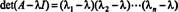Substituting λ = 0 into this identity gives the desired result: det A1, λ 2 … λ n .

If 0 is an eigenvalue of a matrix A, then the equation A x = λ x = 0 x = 0 must have nonzero solutions, which are the eigenvectors associated with λ = 0. But if A is square and A x = 0 has nonzero solutions, then A must be singular, that is, det A must be 0. This observation establishes the following fact: Zero is an eigenvalue of a matrix if and only if the matrix is singular.

Example 3: Determine the eigenvalues and eigenvectors of the identity matrix I without first calculating its characteristic equation.

The equation A x = λ x characterizes the eigenvalues and associated eigenvectors of any matrix A. If A = I, this equation becomes x = λ x. Since x ≠ 0, this equation implies λ = 1; then, from x = 1 x, every (nonzero) vector is an eigenvector of I. Remember the definition: x is an eigenvector of a matrix A if A x is a scalar multiple of x and x ≠ 0. Since multiplication by I leaves x unchanged, every (nonzero) vector must be an eigenvector of I, and the only possible scalar multiple—eigenvalue—is 1.

Example 4: The Cayley‐Hamilton Theorem states that any square matrix satisfies its own characteristic equation; that is, if A has characteristic polynomial p(λ), then p(A) = 0. To illustrate, consider the matrixfrom Example 1. Since its characteristic polynomial is p(λ) = λ 2+3λ+2, the Cayley‐Hamilton Theorem states that p(A) should equal the zero matrix, 0. This is verified as follows:If A is an n by n matrix, then its characteristic polynomial has degree n. The Cayley‐Hamilton Theorem then provides a way to express every integer power A k in terms of a polynomial in A of degree less than n. For example, for the 2 x 2 matrix above, the fact that A 2 + 3 A + 2 I = 0 implies A 2 = −3 A − 2 I. Thus, A 2 is expressed in terms of a polynomial of degree 1 in A. Now, by repeated applications, every positive integer power of this 2 by 2 matrix A can be expressed as a polynomial of degree less than 2. To illustrate, note the following calculation for expressing A 5 in term of a linear polynomial in A; the key is to consistently replace A 2 by −3 A − 2 I and simplify: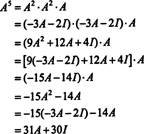This result yields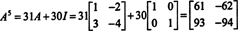a calculation which you are welcome to verify be performing the repeated multiplications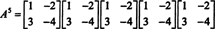The Cayley‐Hamilton Theorem can also be used to express the inverse of an invertible matrix A as a polynomial in A. For example, for the 2 by 2 matrix A above,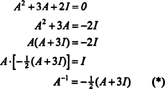This result can be easily verified. The inverse of an invertible 2 by 2 matrix is found by first interchanging the entries on the diagonal, then taking the opposite of the each off‐diagonal entry, and, finally, dividing by the determinant of A. Since det A = 2,but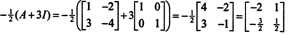validating the expression in (*) for A −1. The same ideas used to express any positive integer power of an n by n matrix A in terms of a polynomial of degree less than n can also be used to express any negative integer power of (an invertible matrix) A in terms of such a polynomial.

Example 5: Let A be a square matrix. How do the eigenvalues and associated eigenvectors of A 2 compare with those of A? Assuming that A is invertible, how do the eigenvalues and associated eigenvectors of A −1 compare with those of A?

Let λ be an eigenvalue of the matrix A, and let x be a corresponding eigenvector. Then A x = λ x, and it follows from this equation thatTherefore, λ 2 is an eigenvalue of A 2, and x is the corresponding eigenvector. Now, if A is invertible, then A has no zero eigenvalues, and the following calculations are justified:so λ −1 is an eigenvalue of A −1 with corresponding eigenvector x.